# NCERT Solutions for Class 9 Science chapter 12 - Sound - eSaralHey, are you a class 9 student and looking for ways to download NCERT Solutions for Class 9 Science Chapter 12 "Sound "? If yes. Then read this post till the end.

In this article, we have listed NCERT solutions for class 9 Science Physics Chapter 12 in PDF that is prepared by Kota’s top IITian’s Faculties by keeping Simplicity in mind.

If you want to learn and understand class 9 Science chapter 12 "Sound " in an easy way then you can use these solutions PDF.

NCERT Solutions helps students to Practice important concepts of subjects easily. Class 9 Science solutions provide detailed explanations of all the NCERT exercise questions that students can use to clear their doubts instantly.

If you want to score high in your class 9 Science Exam then it is very important for you to have a good knowledge of all the important topics, so to learn and practice those topics you can use eSaral NCERT Solutions.

So, without wasting more time Let’s start.

### Download The PDF of NCERT Solutions for Class 9 Science Chapter 12 "Sound"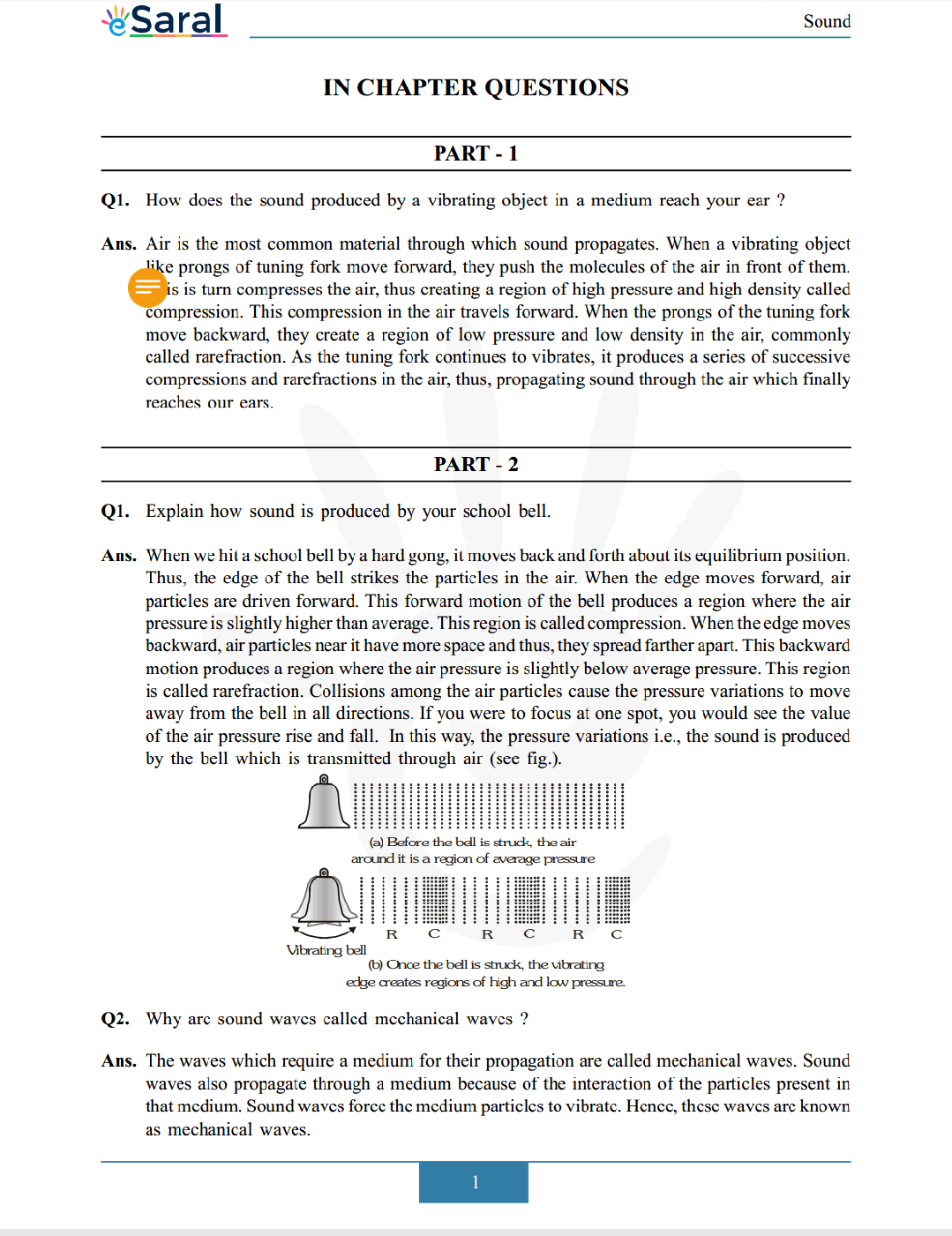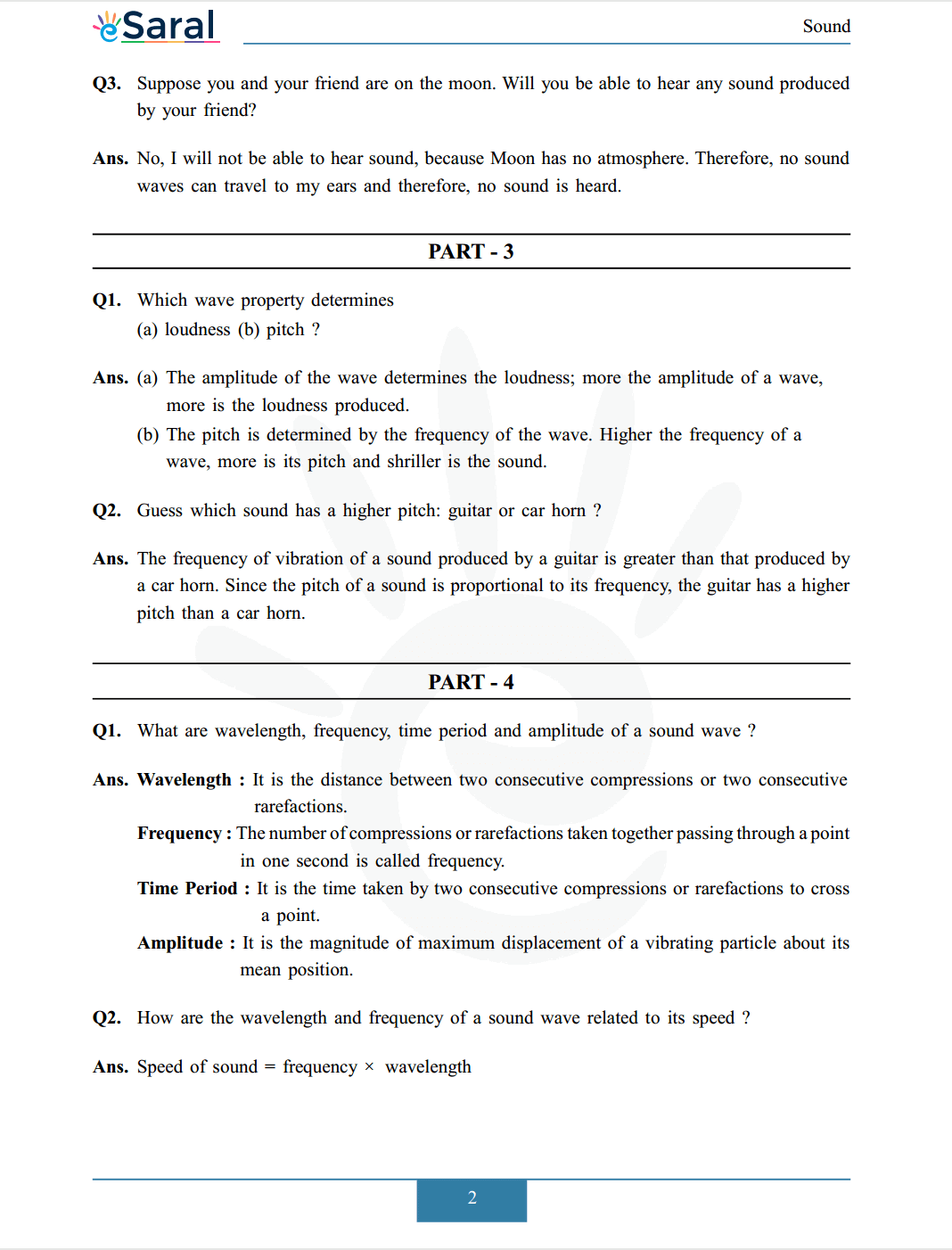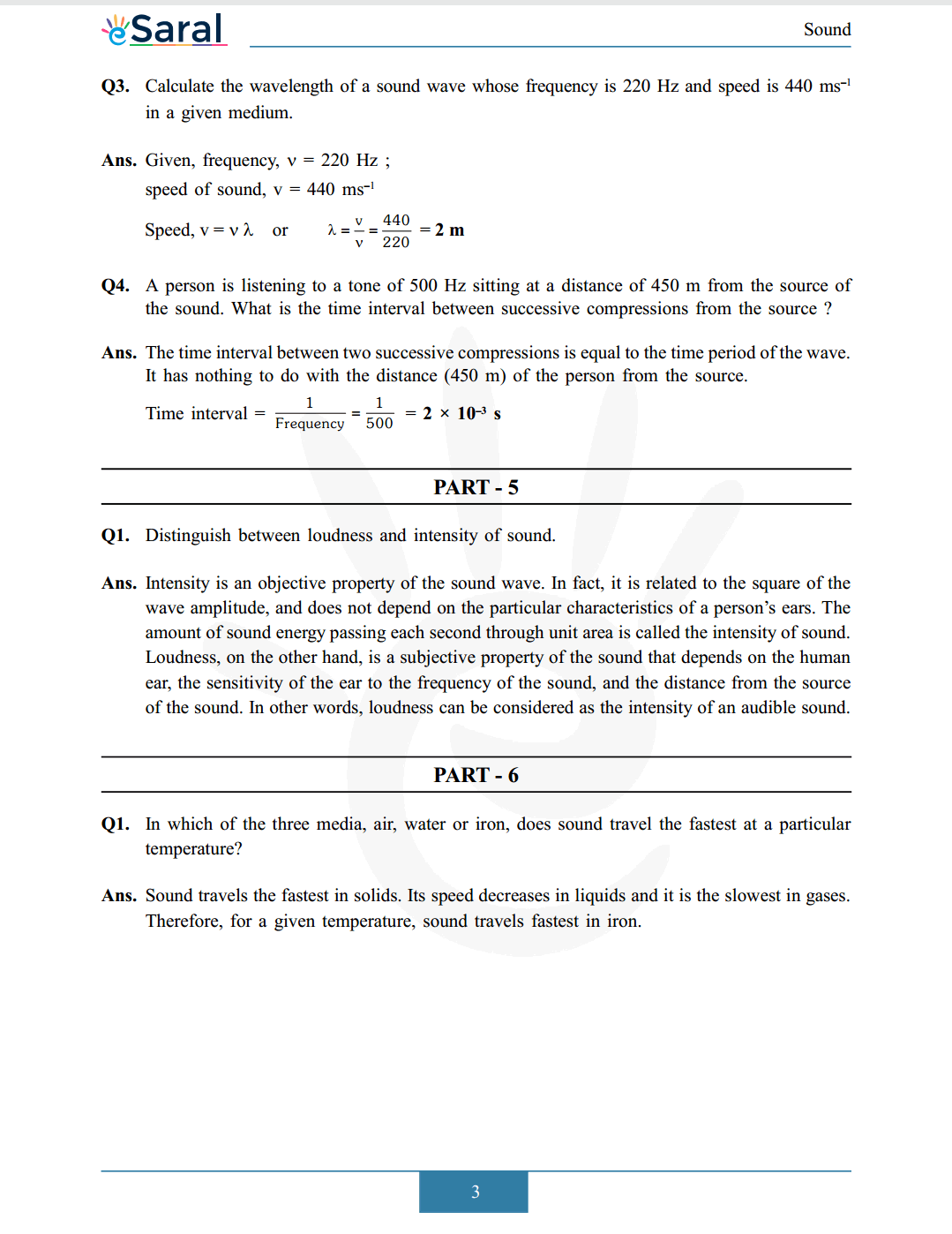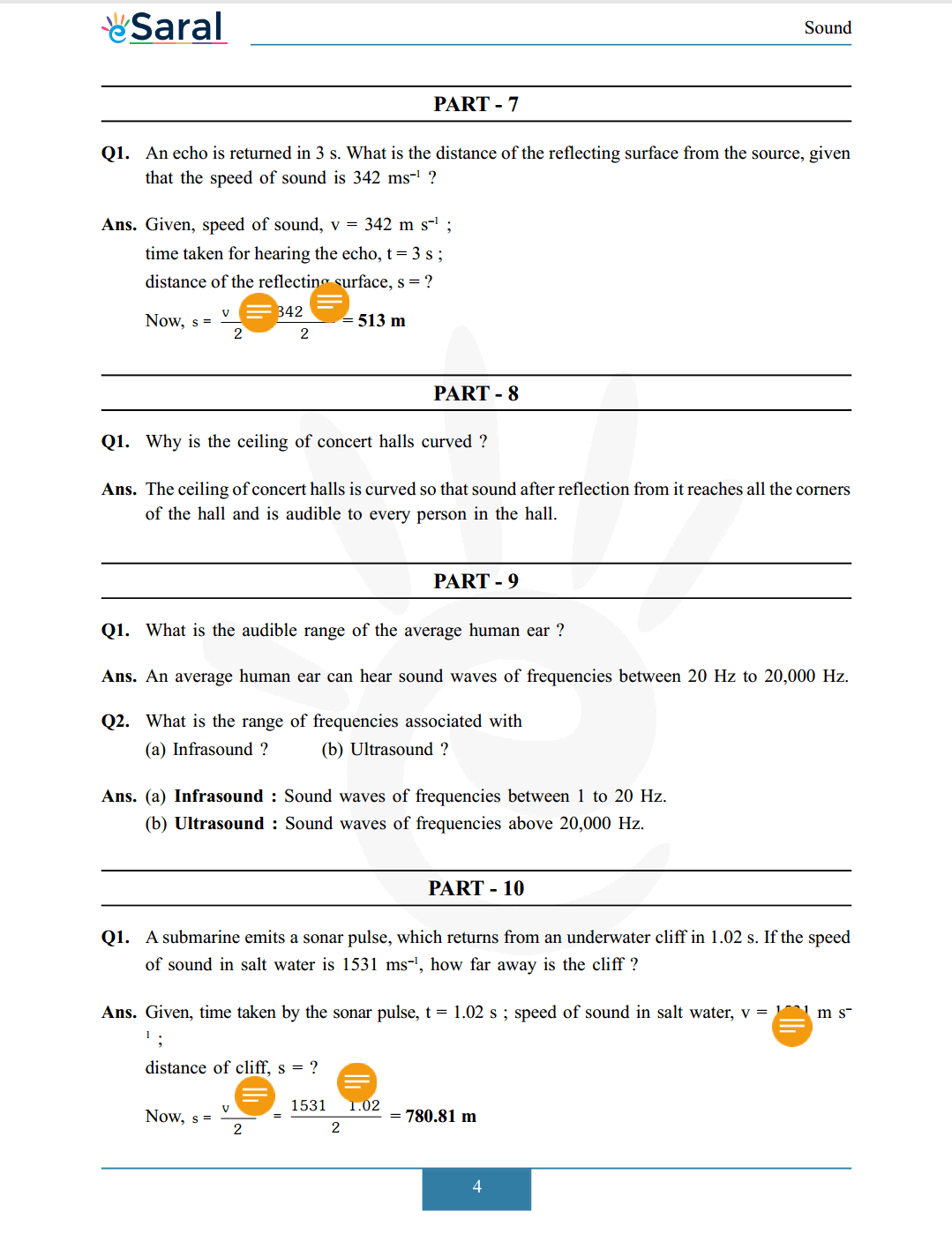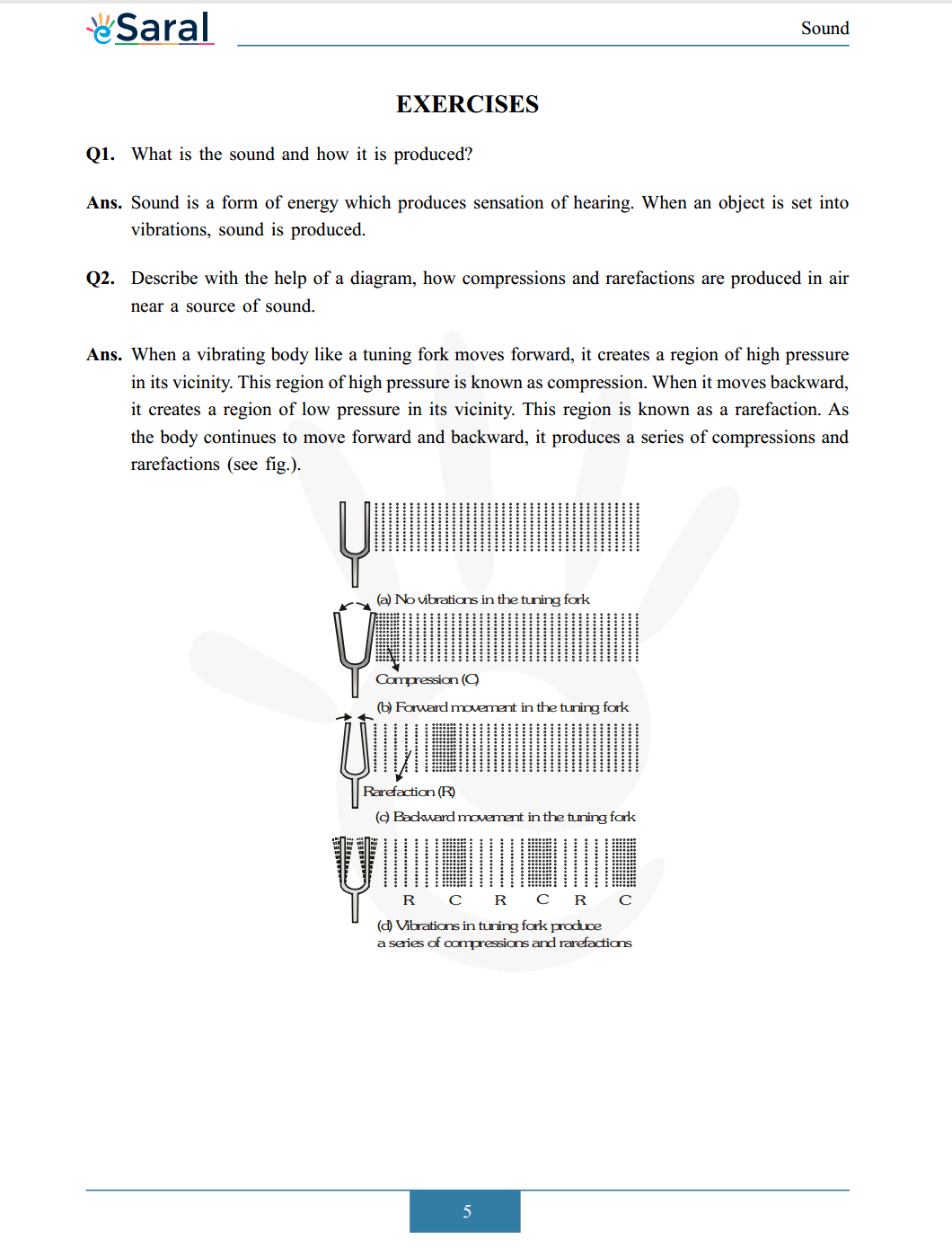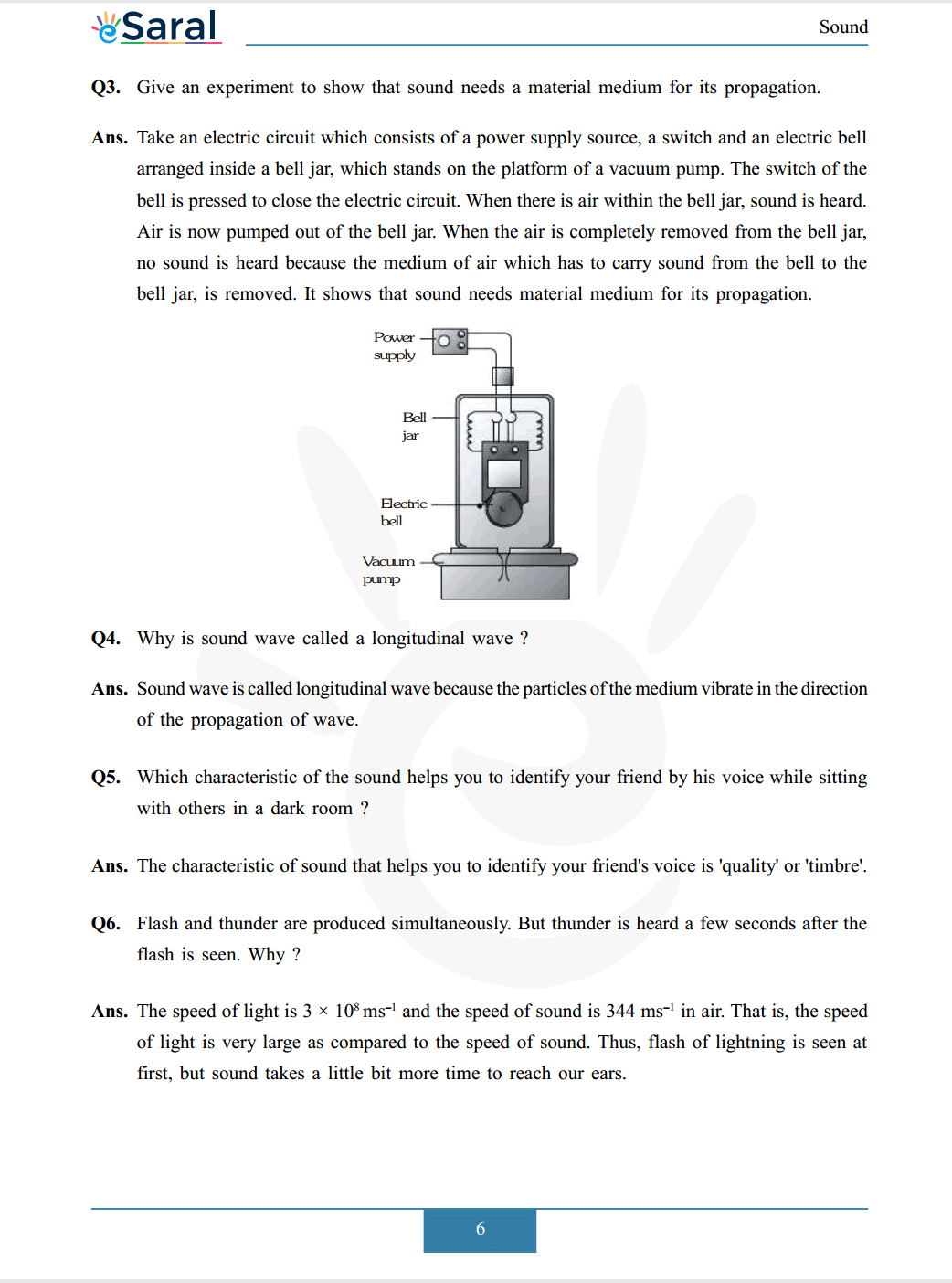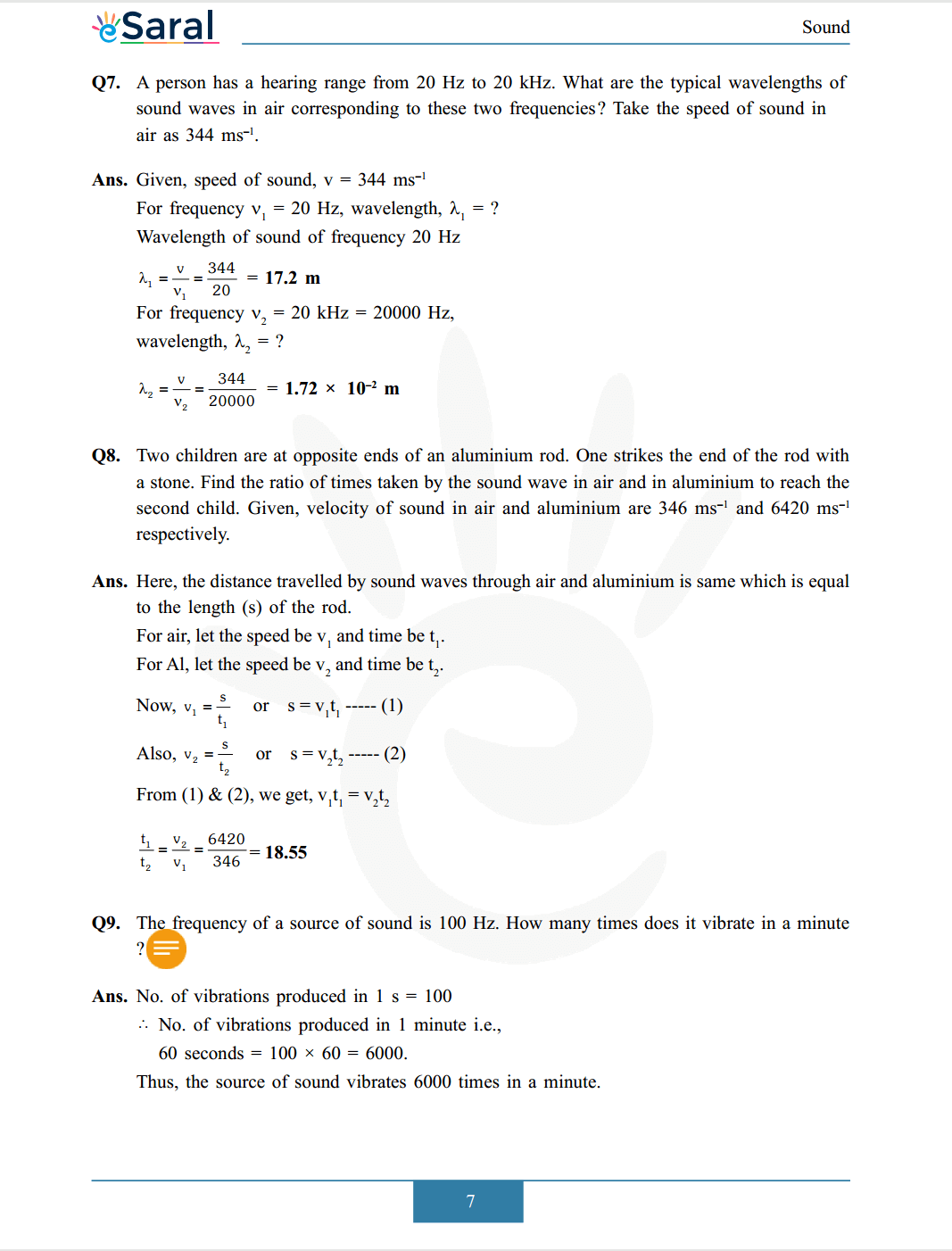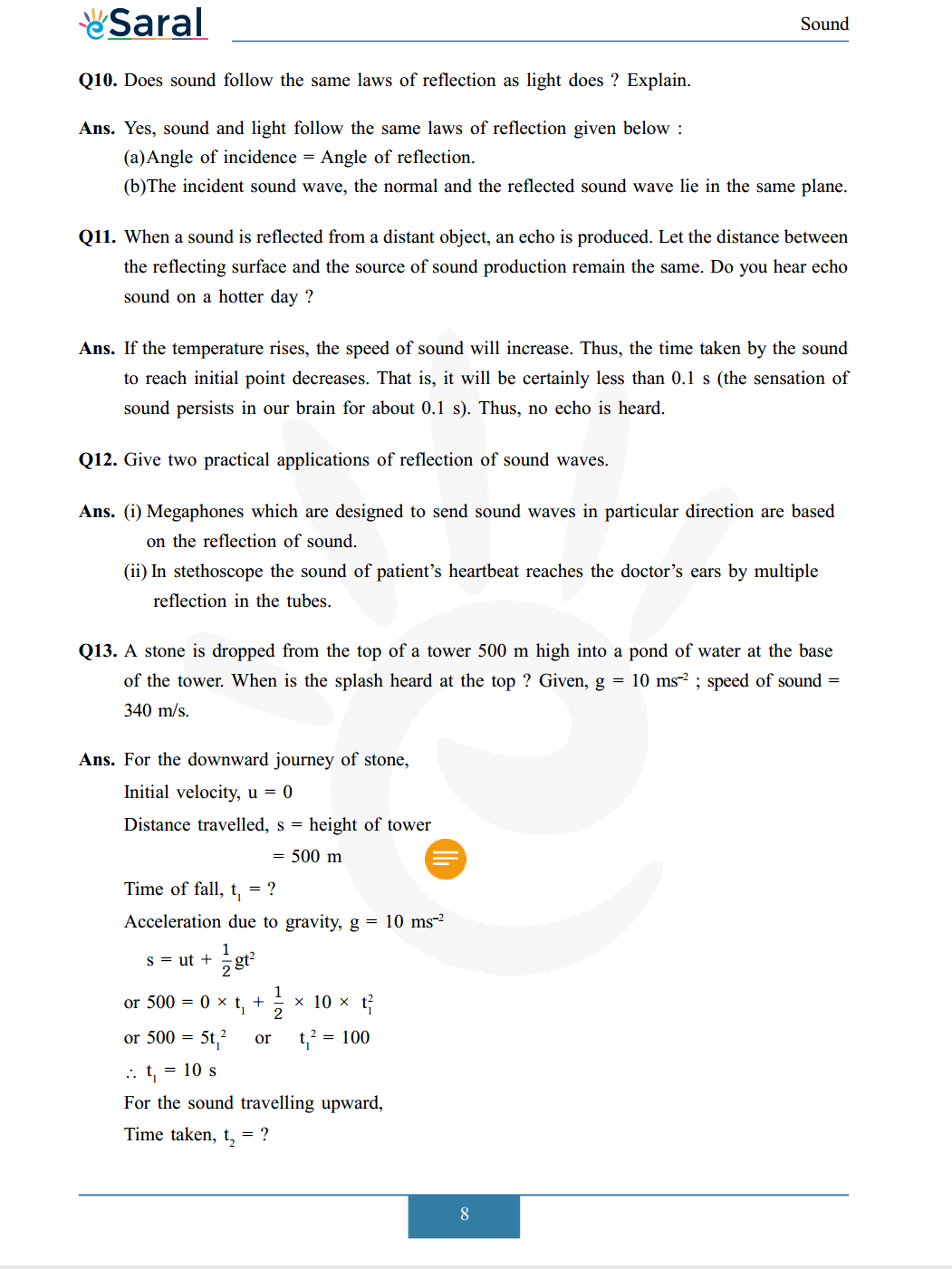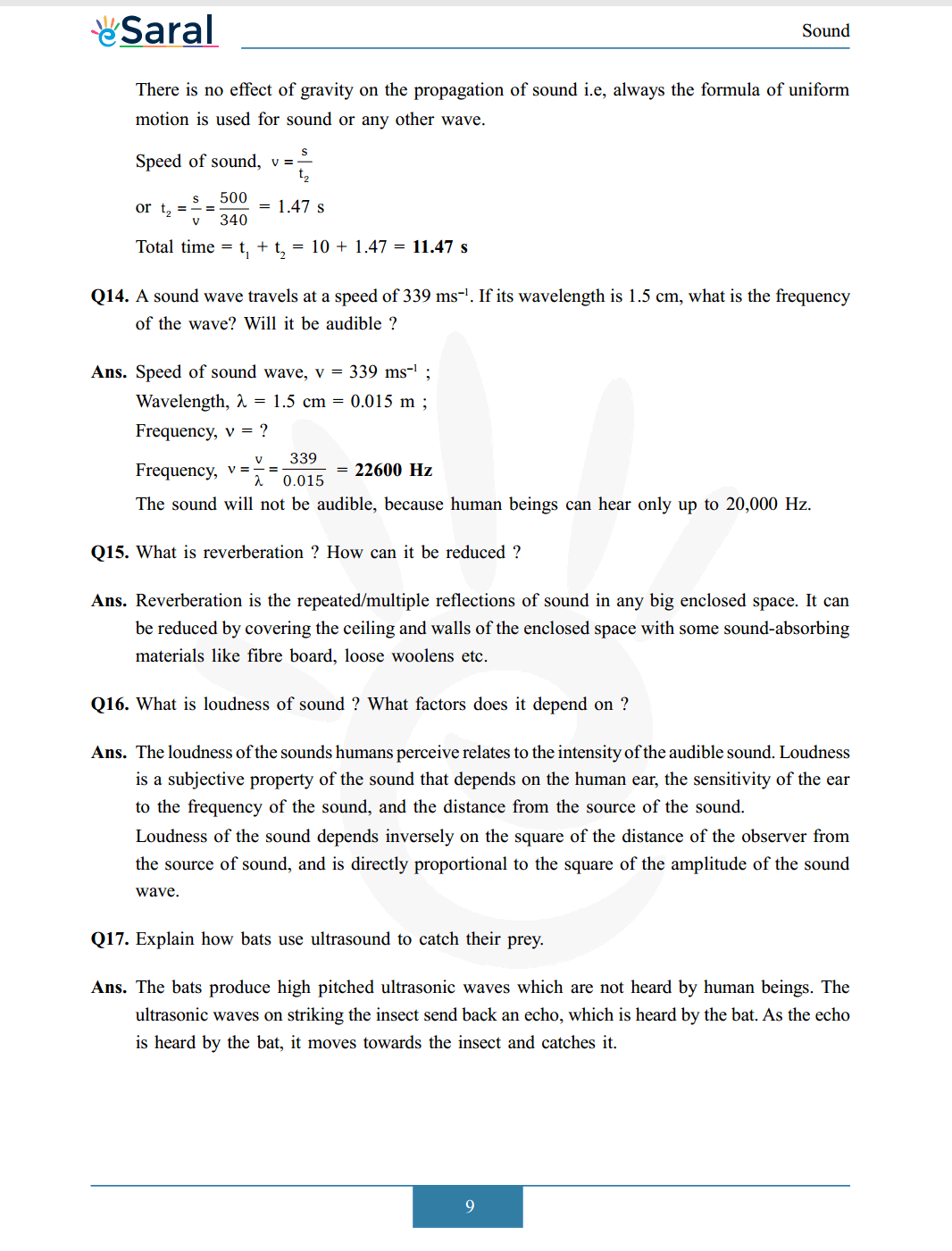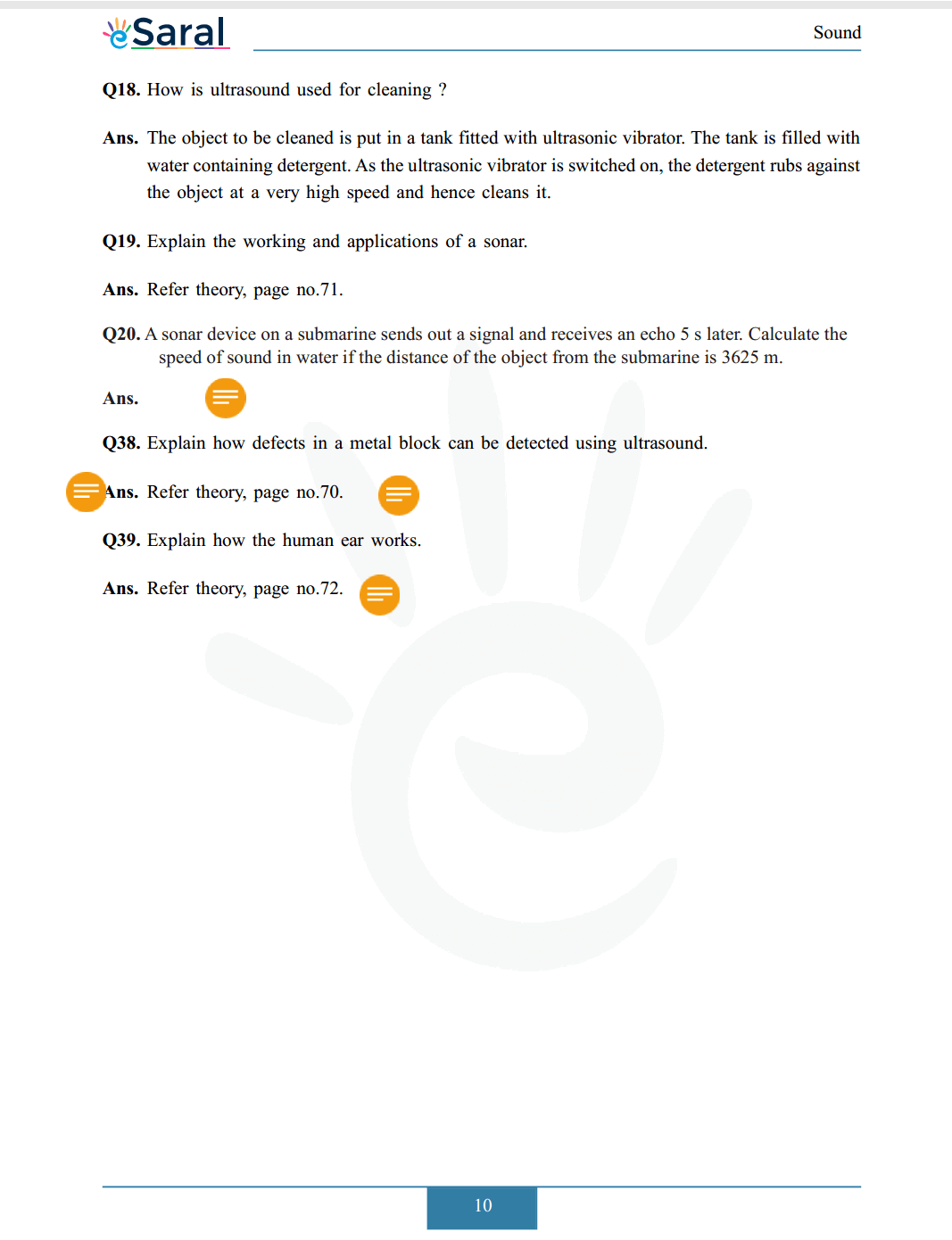Download The PDF of NCERT Solutions for Class 9 Science Chapter 12 "Sound"

### Important topics of class 9 Science Chapter 12

• Sound
• Propagation of Sound
• Reflection of Sound
• Applications of Ultrasound
• Structure of Ultrasound
• Structure of Human Ear
• Production of Sound

#### All Questions of Chapter 12

Once you complete the chapter 12 then you can revise it by solving following questions.

Q1. How does the sound produced by a vibrating object in a medium reach your ear?

##### Part 2

Q1. Explain how sound is produced by your school bell.

Q2. Why are sound waves called mechanical waves?

Q3. Suppose you and your friend are on the moon. Will you be able to hear any sound produced by your friend?

##### Part 3

Q1. Which wave property determines
(a) loudness
(b) pitch?

Q2. Guess which sound has a higher pitch: guitar or car horn?

##### Part 4

Q1. What are wavelength, frequency, time period and amplitude of a sound wave?

Q2. How are the wavelength and frequency of a sound wave related to its speed?

Q3. Calculate the wavelength of a sound wave whose frequency is $220 \mathrm{~Hz}$ and speed is $440 \mathrm{~ms}^{-1}$ in a given medium.

Q4. A person is listening to a tone of $500 \mathrm{~Hz}$ sitting at a distance of $450 \mathrm{~m}$ from the source of the sound. What is the time interval between successive compressions from the source?

##### PART - 5

Q1. Distinguish between loudness and intensity of sound.

##### PART – 6

Q1. In which of the three media, air, water or iron, does sound travel the fastest at a particular temperature?

##### PART – 7

Q1. An echo is returned in $3 \mathrm{~s}$. What is the distance of the reflecting surface from the source, given that the speed of sound is $342 \mathrm{~ms}^{-1}$ ?

##### PART – 8

Q1. Why is the ceiling of concert halls curved?

##### PART – 9

Q1. What is the audible range of the average human ear?

Q2. What is the range of frequencies associated with?
(a) Infrasound?
(b) Ultrasound?

##### PART – 10

Q1. A submarine emits a sonar pulse, which returns from an underwater cliff in $1.02 \mathrm{~s}$. If the speed of sound in salt water is $1531 \mathrm{~ms} ^{-1}$, how far away is the cliff ?

##### EXERCISES

Q1. What is the sound and how it is produced?

Q2. Describe with the help of a diagram, how compressions and rarefactions are produced in air near a source of sound.

Q3. Give an experiment to show that sound needs a material medium for its propagation.

Q4. Why is sound wave called a longitudinal wave?

Q5. Which characteristic of the sound helps you to identify your friend by his voice while sitting with others in a dark room?

Q6. Flash and thunder are produced simultaneously. But thunder is heard a few seconds after the flash is seen. Why?

Q7. A person has a hearing range from $20 \mathrm{~Hz}$ to $20 \mathrm{kHz}$. What are the typical wavelengths of sound waves in air corresponding to these two frequencies? Take the speed of sound in air as $344 \mathrm{~ms}^{-1}$.

Q8. Two children are at opposite ends of an aluminium rod. One strikes the end of the rod with a stone. Find the ratio of times taken by the sound wave in air and in aluminium to reach the second child. Given, velocity of sound in air and aluminium are $346 \mathrm{~ms}^{-1}$ and $6420 \mathrm{~ms}^{-1}$ respectively.

Q9. The frequency of a source of sound is $100 \mathrm{~Hz}$. How many times does it vibrate in a minute?

Q10. Does sound follow the same laws of reflection as light does? Explain.

Q11. When a sound is reflected from a distant object, an echo is produced. Let the distance between the reflecting surface and the source of sound production remain the same. Do you hear echo sound on a hotter day?

Q12. Give two practical applications of reflection of sound waves.

Q13. A stone is dropped from the top of a tower $500 \mathrm{~m}$ high into a pond of water at the base of the tower. When is the splash heard at the top? Given, $\mathrm{g}=10 \mathrm{~ms}^{-2} ;$ speed of sound $=$ $340 \mathrm{~m} / \mathrm{s}$

Q14. A sound wave travels at a speed of $339 \mathrm{~ms}^{-1}$. If its wavelength is $1.5 \mathrm{~cm}$, what is the frequency of the wave? Will it be audible?

Q15. What is reverberation? How can it be reduced?

Q16. What is loudness of sound? What factors does it depend on?

Q17. Explain how bats use ultrasound to catch their prey.

Q18. How is ultrasound used for cleaning?

Q19. Explain the working and applications of a sonar.

Q20. A sonar device on a submarine sends out a signal and receives an echo 5 s later. Calculate the speed of sound in water if the distance of the object from the submarine is 3625 m.

Q38. Explain how defects in a metal block can be detected using ultrasound.

Q39. Explain how the human ear works.